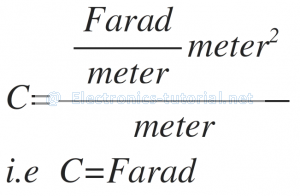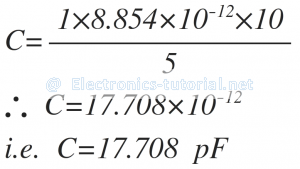Home > electronic components > capacitors > parallel plate capacitor

# Parallel Plate Capacitor

In parallel plate capacitor the two metallic plates are separated by the dielectric material which is made up plastic, wax paper, mica etc. Sometime peoples also create the air gap between the two plates. Hence, in this case the air acts as the dielectric between the two plates of the capacitor. This airgap is nothing but the vacuum created between the two metallic plates. Figure below shows the parallel plate capacitor.From the figure it can be seen that, the capacitance of the parallel plate capacitor is depends upon cross-sectional area of the metallic plate i.e. “A” in meter^2, the distance between the two metallic plates i.e. “d” in meter and the dielectric constant of the material used in between the two plates i.e. εr. The parameter εr is also called as relative permittivity of the material. The εr value for the free space or air or vacuum is unity i.e. 1.
The parallel plate capacitor is given by the following equation.Where, ε0 is the permittivity of the air which has the constant value i.e. 8.854 ×10 ^-12 Farads/meter.
Thus the unit of Capacitance is given by,Sample Problem
1) If A=10 m2, d=5m, dielectric is air.
As we know that, The capacitance is given by,Here, dielectric is air.
Therefore, εr= 1.